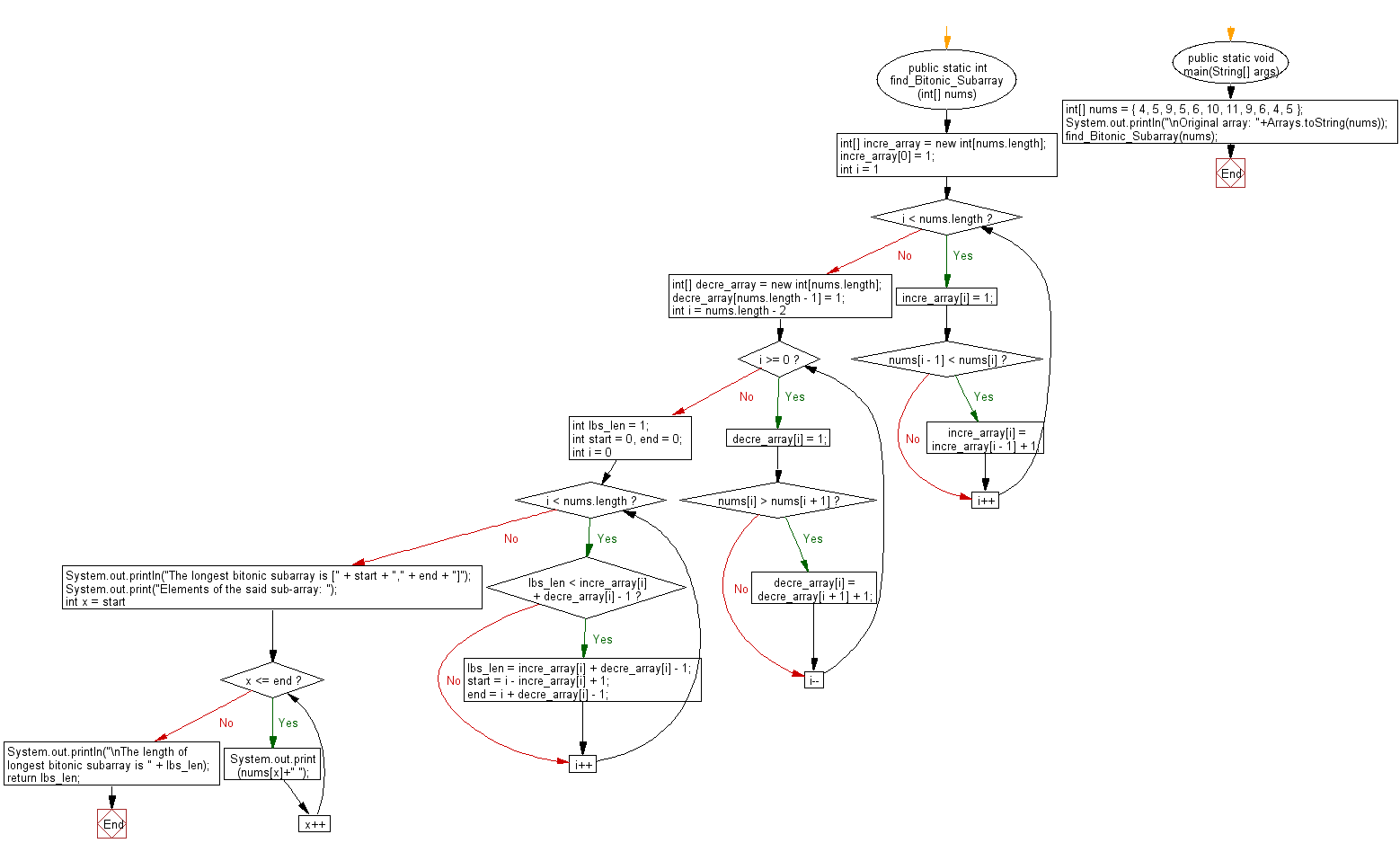﻿ Java exercises: Find Longest Bitonic Subarray in a given array - w3resource# Java Array Exercises: Find Longest Bitonic Subarray in a given array

## Java Array: Exercise-64 with Solution

Write a Java program to find Longest Bitonic Subarray in a given array.

A bitonic subarray is a subarray of a given array where elements are first sorted in increasing order, then in decreasing order. A strictly increasing or strictly decreasing subarray is also accepted as bitonic subarray.

Example:
Input :
nums = { 4, 5, 9, 5, 6, 10, 11, 9, 6, 4, 5 }
Output:
The longest bitonic subarray is [3,9]
Elements of the said sub-array: 5 6 10 11 9 6 4
The length of longest bitonic subarray is 7

Sample Solution:

Java Code:

``````import java.util.Arrays;
class solution
{
public static int find_Bitonic_Subarray(int[] nums)
{
int[] incre_array = new int[nums.length];
incre_array = 1;
for (int i = 1; i < nums.length; i++) {
incre_array[i] = 1;
if (nums[i - 1] < nums[i]) {
incre_array[i] = incre_array[i - 1] + 1;
}
}

int[] decre_array = new int[nums.length];
decre_array[nums.length - 1] = 1;
for (int i = nums.length - 2; i >= 0; i--) {
decre_array[i] = 1;
if (nums[i] > nums[i + 1]) {
decre_array[i] = decre_array[i + 1] + 1;
}
}

int lbs_len = 1;
int start = 0, end = 0;

for (int i = 0; i < nums.length; i++)
{
if (lbs_len < incre_array[i] + decre_array[i] - 1)
{
lbs_len = incre_array[i] + decre_array[i] - 1;
start = i - incre_array[i] + 1;
end = i + decre_array[i] - 1;
}
}

// print longest bitonic sub-array
System.out.println("The longest bitonic subarray is [" + start + "," + end + "]");
System.out.print("Elements of the said sub-array: ");
for (int x = start; x <= end; x++)
{

System.out.print(nums[x]+" ");
}

System.out.println("\nThe length of longest bitonic subarray is " + lbs_len);

return lbs_len;
}

public static void main(String[] args)
{
int[] nums = { 4, 5, 9, 5, 6, 10, 11, 9, 6, 4, 5 };
System.out.println("\nOriginal array: "+Arrays.toString(nums));
find_Bitonic_Subarray(nums);
}
}
```
```

Sample Output:

```Original array: [4, 5, 9, 5, 6, 10, 11, 9, 6, 4, 5]
The longest bitonic subarray is [3,9]
Elements of the said sub-array: 5 6 10 11 9 6 4
The length of longest bitonic subarray is 7
```

Flowchart:Java Code Editor:

Improve this sample solution and post your code through Disqus

What is the difficulty level of this exercise?

Test your Programming skills with w3resource's quiz.

﻿

## Java: Tips of the Day

countOccurrences

Counts the occurrences of a value in an array.

Use Arrays.stream().filter().count() to count total number of values that equals the specified value.

```public static long countOccurrences(int[] numbers, int value) {
return Arrays.stream(numbers)
.filter(number -> number == value)
.count();
}
```

Ref: https://bit.ly/3kCAgLb